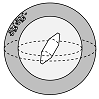# Suborbital Transit with Hovering

The mathematically optimal solution for the lowest-fuel trajectory to drive a rocket from one point on a planetary body to another (in vacuum) is a question that is easily settled. A simple ballistic trajectory (an elliptical orbit) that goes from point A to point B with the apogee in the center of the two will do the job.

In the real world, however, we maximize profits, not propellant.

## Fully Ballistic (and Deadly) Trips

This fascinated me as a criticism of SpaceX’s idea for commercial passenger transport. Some details are here:

Practically, no one would ever do this, of course. That left me with a nasty nagging question — what kind of trajectories would it be reasonable for us to assume commercial suborbital travel would take? Would any trip require reaching orbital velocity? That seems absurd. For proof by absurdity, surely you do not need to reach orbital velocity to fly (on a rocket) from your current location to the next room over. No, you would accept some additional gravity drag and hover carefully.

Logically, some cross-over must happen, and it’s non-obvious whether long-distance trips would benefit from something like mid-trip upwards thrust (so that it need not reach such a high velocity). So I set about to find out.

## Semi-Orbital with Hovering

The trip still goes in an arc, just like you would if taking it at full orbital velocity. However, you have some smaller engine that maintains a continuous upwards thrust through the stages of flight that would have otherwise been zero-gravity coasting. The general constraints are outlined below. The first constraint is that the coasting phase must maintain a constant altitude, so the upward thrust plus the rotational acceleration must balance gravity. The second constraint is that the trip Delta V value must be at a minimum.

This is pretty much enough information to churn through the equations, and I did that here. I’m taking the variable theta to be in radians.

This is a strange strange outcome. If theta is 1/2 you find that it’s a wash — there is no longer any benefit to the scheme at distances further than this.

Here I generated a plot, and plugged in some actual numbers.theta is the angle between point A and point B, and the fraction relates to the fuel saved

What the heck does this mean for distances greater than the maximum here? In the math above, I took the derivative as a means of finding a local minimum for Delta V. Above theta=1.0, it would appear that the slope of the function just means that it costs more fuel for any amount of hovering.

For some practicality, a value of 1.0 means 1.0 radians, measured as an angle about the center of the Earth. In terms of surface distance, this is about 1981 miles. In terms of distance from New York, NY, it is the following:

Bottom line: mid-filght hovering has a benefit for trips from New York, NY to all trips inside of the blue circle, but the benefit mostly matters for trips to places closest to the center of the circle.

If you go from New York to LA, then the most optimal way to get there with a rocket is to just achieve orbital velocity, assuming you have ruled out the ballistic trajectory option. Not so for New York to Dallas. Who knew?

## Another Option — Propulsive Slowing

That option strikes me as much more mathematically demanding.

--

--Obligatory analytical writing, online participation account for Medium. Engineering, software, books, space, constant daydreaming.